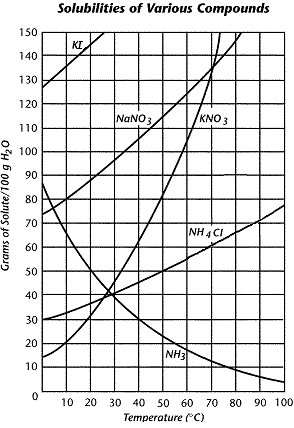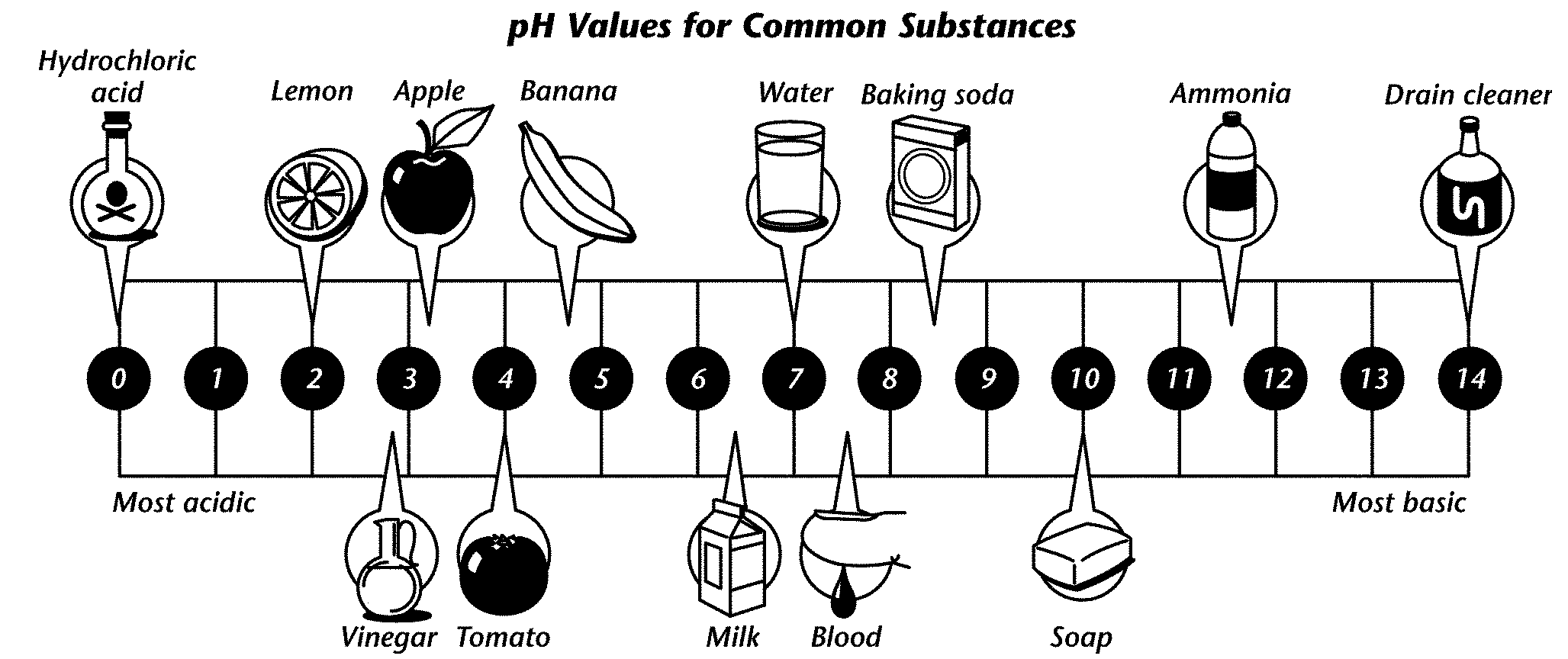# Chapter 7 review Completion

Download 90,26 Kb.
 Date conversion 04.06.2017 Size 90,26 Kb.
 Chapter 7 review Completion Complete each statement. 1. A(n) ____________________ is a mixture that appears uniform throughout and whose particles cannot easily be separated. 2. Because it will dissolve so many solutes, ____________________ is sometimes called the universal solvent. 3. Ionic and polar compounds ____________________ in water because water molecules are polar. 4. If you can continue to add more solute to a solution, the solution is said to be ____________________. 5. A(n) ____________________ is a compound that tastes sour and reacts with some metals. 6. A(n) ____________________ is a compound that turns litmus blue and is often found in soaps and detergents. 7. Acids are ____________________, which means that they “eat away” at other materials. 8. Acids and bases have many uses around the ____________________ and in industry. 9. Substances that form hydrogen ions when released in water are called ____________________. 10. When the pH is high, the concentration of hydrogen ions is ____________________. 11. At the same concentrations, strong acids produce more ____________________ than weak acids. 12. In a reaction of an acid with a base, the pH changes to a value that is closer to ____________________. 13. A(n) ____________________ is an ionic compound produced from the neutralization of an acid with a base. 14. A(n) ____________________ solution has more dissolved solute than is predicted by its solubility at a given temperature. 15. Mayonnaise, whipped cream, and milk are examples of mixtures known as ____________________. 16. An atom of hydrogen that has lost its electron is called a(n) ____________________. Short Answer Use the diagram to answer each question.17. What happens to the solubility of potassium nitrate (KNO3) as the temperature rises? 18. According to the graph, which of the compounds is most soluble at 0°C? Which is least soluble at 100°C? 19. Compare the solubility of sodium nitrate (NaNO3) to that of ammonia (NH3). 20. About how many grams of potassium nitrate (KNO3) will settle out when a saturated solution made in 100 grams of water is cooled from 70°C to 50°C? 21. Above 70°C, what other compounds besides ammonia (NH3) have a lower solubility than that of potassium nitrate (KNO3)? 22. At 70°C, 60 grams of ammonium chloride (NH4Cl) are added to 100 grams of water. What will happen if 10 grams more of ammonium chloride are added to the solution? Explain. Use the diagram to answer each question.23. Use the pH scale to compare an apple and a lemon. 24. Why would you expect soap to taste bitter? 25. What does the pH of drain cleaner tell you about the dangers of such a product? 26. What color would litmus paper turn in a solution of baking soda? Explain your answer in terms of pH. 27. What does the pH of water tell you about how the water differs from the other items shown in the diagram? 28. In terms of pH, explain what would happen if you mixed vinegar with a solution of ammonia. What type of products would form? Essay 29. Describe the differences among solutions, colloids, and suspensions. 30. Describe how particles of table salt (an ionic compound) and table sugar (a molecular compound) differ in the way they dissolve in water. 31. Explain why the coolant (antifreeze in water) in a car’s radiator protects the engine from damage. 32. You are given three clear, colorless, unknown solutions, and red and blue litmus papers. Describe the steps you might take to correctly identify each solution as acidic, basic, or neutral. 33. Describe how solutions of acids and bases differ in terms of particles. How do the differences relate to the properties of acids and bases? 34. Describe the neutralization process and give an example of the reaction using hydrochloric acid (HCl) and potassium hydroxide (KOH). 35. Your teacher sends you to the lab to retrieve a jar of salt and a jar of sugar. You find two jars containing white powder, but you aren't sure which one contains salt and which one contains sugar. Since the substances were found in a laboratory, tasting them is unsafe. What else can you do to determine which substance is salt and which one is sugar? Explain your reasoning. 36. Explain why the directions for cooking pasta often advise adding salt to the water.

The database is protected by copyright ©sckool.org 2016
send message